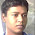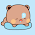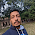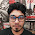Tuesday, December 8, 2009

Recursions are fun

You may like to try out some simple problems to practice recursions. Try to solve all of them without using any global variables. And try on your own before looking at the solutions. Also please notify any error to me ( zobayer1[at]gmail[dot]com ).

Before looking at the problems, you may like to read this post about how to attack recursive problems.

Problem 1:

You will be given an array of integers, write a recursive solution to print it in reverse order.
`Input:569 87 45 21 47Output:47 21 45 87 69`

Problem 2:

Write a recursive function to print an array in the following order.
 [n-1]
 [n-2]
.........
.........
[(n-1)/2] [n/2]
`Input:51 5 7 8 9Output:1 95 87 7`

Problem 3:

Write a recursive program to remove all odd integers from an array. You must not use any extra array or print anything in the function. Just read input, call the recursive function, then print the array in main().
`Input:61 54 88 6 55 7Output:54 88 6`

Problem 4:

Write a recursive solution to print the polynomial series for any input n:
1 + x + x2 + ................. + xn-1
`Input:5Output:1 + x + x^2 + x^3 + x^4`

Problem 5:

Write a recursive solution to evaluate the previous polynomial for any given x and n.
Like, when x=2 and n=5, we have 1 + x + x2 + ................. + xn-1 = 31
`Input:2 5Output:31`

Problem 6:

Write a recursive program to compute n!
`Input:5Output:120`

Problem 7:

Write a recursive program to compute nth fibonacci number. 1st and 2nd fibonacci numbers are 1, 1.
`Input:6Output:8`

Problem 8:

Write a recursive program to determine whether a given integer is prime or not.
`Input:499999831Output:not primeprimenot prime`

Problem 9:

Write a recursive function that finds the gcd of two non-negative integers.
`Input:25 8895Output:5`

Problem 10:

Write a recursive solution to compute lcm of two integers. You must not use the formula lcm(a,b) = (a x b) / gcd(a,b); find lcm from scratch...
`Input:23 488Output:11224`

Problem 11:

Suppose you are given an array of integers in an arbitrary order. Write a recursive solution to find the maximum element from the array.
`Input:57 4 9 6 2Output:9`

Problem 12:

Write a recursive solution to find the second maximum number from a given set of integers.
`Input:55 8 7 9 3Output:8`

Problem 13:

Implement linear search recursively, i.e. given an array of integers, find a specific value from it.
Input format: first n, the number of elements. Then n integers. Then, q, number of query, then q integers. Output format: for each of the q integers, print its index (within 0 to n-1) in the array or print 'not found', whichever is appropriate.
`Input:52 9 4 7 625 9Output:not found1`

Problem 14:

Implement binary search recursively, i.e. given an array of sorted integers, find a query integer from it.
Input format: first n, the number of elements. Then n integers. Then, q, number of query, then q integers. Output format: for each of the q integers, print its index (within 0 to n-1) in the array or print 'not found', whichever is appropriate.
`Input:51 2 3 4 523 -5Output:2not found`

Problem 15:

Write a recursive solution to get the reverse of a given integer. Function must return an int
`Input:123405Output:504321`

Problem 16:

Read a string from keyboard and print it in reversed order. You must not use any array to store the characters. Write a recursive solutions to solve this problem.
`Input:hellooOutput:oolleh`

Problem 17:

Write a recursive program that determines whether a given sentence is palindromic or not just considering the alpha-numeric characters ('a'-'z'), ('A'-'Z'), ('0'-'9').
`Input:madam, i'm adamhulalaOutput:palindromicnot palindromic`

Problem 18:

Implement strcat(), stracpy(), strcmp() and strlen() recursively.
`Input:test on your ownOutput:test on your own`

Problem 19:

If you already solved the problem for finding the nth fibonacci number, then you must have a clear vision on how the program flow works. So now, in this problem, print the values of your fibonacci function in pre-order, in-order and post-order traversal. For example, when n = 5, your program calls 3 and 4 from it, from the call of 3, your program calls 1 and 2 again....... here is the picture:`Input:5Output:Inorder: 1 3 2 5 2 4 1 3 2Preorder: 5 3 1 2 4 2 3 1 2Postorder: 1 2 3 2 1 2 3 4 5`

Problem 20:

All of you have seen the tower of Hanoi. You have 3 pillars 'a', 'b' and 'c', and you need transfer all disks from one pillar to another. Conditions are, only one disk at a time is movable, and you can never place a larger disk over a smaller one. Write a recursive solution to print the moves that simulates the task, a -> b means move the topmost of tower a to tower b.
`Input:3Output:a -> ca -> bc -> ba -> cb -> ab -> ca -> c`

Good Luck!!!

1.really nice :)

2.need some critical recursion?

3.Haha, I have plenty :) I think these are enough for beginners to give them a head start, after these, they can practice on their own.

But If you think that you can help them further, you can post here the problems, or source links or whatever you have.

4.Truly Helpful Brother...May Allah Bless you :)

5.ইনফিনিটিভ(অসংখ্য) ধন্যবাদ।অনেক কিছু প্রেক্টিস করতে পারলাম।
সাকিব হাসান,ইবি,কুস্টিয়া,বাংলাদেশ।

1.I am really glad that it helped, my best wishes for you :)

6.I am trying to learn recursion.I can write easy recursion.But when I am trying to write a recursion for backtracking problem or dp, most of the time I failed.But if I see a medium hard recursive func I relaize it easily.But if I try to write a new recusion most of the time I failed.

1.Actually, after solving lots of dp and recursive problem, this is what I would say: If you want to learn dp / backtracking algorithm, you have to solve as many dp as possible, the only way to improve dp skill is to solving more and more. Frustrating at some points, but I don't think there is any other options.

7.wow!! It is very helpful for me and anyone . Thank you so much for this good job.

8.thank u so much vai..............really helpful!

9.That's damn important and fun ........................... Had a lot of fum :D

1.I'm glad that you liked it :)

10.I have tested the following code and it give me correct result But the answer script has one extra line before recursive call. the line is "if(*sbest < a[i]) *sbest = a[i];". Is it necessary? If necessary, would you kindly explain to me.

void sMax(int i, int n, int *a, int *fbest, int *sbest)
{
if (i == n - 1)
{
*fbest = a[i];
return;

}

sMax((i + 1), n, a, fbest, sbest);

if (a[i] > *fbest)
{
*sbest = *fbest;
*fbest = a[i];
}
else if (a[i] > *sbest)
*sbest = a[i];

return;

}

11.কিছু কিছু Problem বুঝতে পারলাম না !

1.12.int main(){
printf("Thank you\n");
main();
}

1.haha, nice recursion, (but your program cannot call main() )
you're welcome!

13.Can u plz tell me what's wrong with my code? All the time, it returns the correct ans with an extra value. why is there an extra?
int call(int i,int n,int a[])
{
if(i<=n)
{

cout<>n;
{
for(int i=0; i>a[i];
cout<<call(0,n-1,a)<<endl;
return 0;
}

}

1.Hi, your code is broken because of HTML characters. Can you post your code here and then give me the link?

14.Thanks so so so so so sooooooooooooooooooooooooooooooooooooooooooo much <3

1.You are welcome :)

15.how i can write a recursive problem to generate all permutations of a given n numbers?

16.//============================================================================
// Name : TowerOfHanoiRecursion.cpp
// Author : Nitish Raj, Scientist, DRDO, raj.nitp@gmail.com
// Version :
// Description : Ansi-style
//============================================================================

#include
#include
#include

#include
using namespace std;

/*
| | |
___|___ __|__ __|__
Source Auxilary Destination

*/
/*Function say whenever you call this second argument will be always from where
you have to element and fourth argument says where you need to put and third used as
spare */

stack A,B,C;

void moveData(char Src, char Des){

switch(Src)
{
case 'a':
{
if(Des == 'b') B.push(A.top());
if(Des == 'c') C.push(A.top());
A.pop();
break;
}
case 'b':
{
if(Des == 'a') A.push(B.top());
if(Des == 'c') C.push(B.top());
B.pop();
break;
}
case 'c':
{
if(Des == 'b') B.push(C.top());
if(Des == 'a') A.push(C.top());
C.pop();
break;
}
}

}
void ShowDataofStack(){
stack temp;
cout<<"TOWER A :: ";
temp = A;

while(!temp.empty()){
cout<0)
{
HanoiTowerRec(n-1, Source,Destination, Aux); //

// Now nth element left on Source so put this to Destination;
cout<<"______________________________________ "<"<>n;
for(int i =0 ; i<n;i++) A.push(n-i);
HanoiTowerRec(n, 'a', 'b', 'c');

return 0;
}

17./**
Write a recursive solution to evaluate the previous polynomial for any given x and n.
Like, when x=2 and n=5, we have 1 + x + x2 + ................. + xn-1 = 31

Input:
2 5
Output:
31

**/

#include
using namespace std;
int print(int i,int x,int n,int sum)
{
if(i==1)
{
sum=1;
}
if(i>1)
{
sum+=pow(x,i-1);
}
if(i==n)
return sum;
print(i+1,x,n,sum);
}
int main()
{
cout<<print(1,2,5,0)<<endl;;
return 0;
}

ভাইয়া আমি এইভাবে করছি। আমারটা কি হইছে? নাকি কোন বাগ আছে? জানালে ভালো হত।

1.Why do you set sum = 1 when i == 1.

18.sakhawatshamim35@gmail.com এই ইমেইল ও জানাতে পারেন।

19.20.problem 12,20 seems very difficult than others .

21.Can anyone tell what is use of return statement while returning a value in recursion...
What if I don't write return in front of function naming which is not of void type?

1.It will raise warning during compilation, also, the method will return a garbage value if any statement that called the function initially was expecting a value. If you do not expect a return value from a function call, then there is not problem. Look at the following example:

int a() {
// do not return anything
}

---- in main ---
a(); // no problem
int x = a(); // now there is a problem

I hope this clears up the confusion.